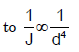Courses

# Torsion - MCQ Test 2

## 30 Questions MCQ Test GATE Mechanical (ME) 2022 Mock Test Series | Torsion - MCQ Test 2

Description
This mock test of Torsion - MCQ Test 2 for Mechanical Engineering helps you for every Mechanical Engineering entrance exam. This contains 30 Multiple Choice Questions for Mechanical Engineering Torsion - MCQ Test 2 (mcq) to study with solutions a complete question bank. The solved questions answers in this Torsion - MCQ Test 2 quiz give you a good mix of easy questions and tough questions. Mechanical Engineering students definitely take this Torsion - MCQ Test 2 exercise for a better result in the exam. You can find other Torsion - MCQ Test 2 extra questions, long questions & short questions for Mechanical Engineering on EduRev as well by searching above.
QUESTION: 1

Solution:
QUESTION: 2

Solution:
QUESTION: 3

### In power transmission shafts, if the polar moment of inertia of a shaft is doubled, then what is the torque required to produce the same angle of twist?

Solution: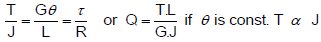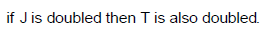QUESTION: 4

For a power transmission shaft transmitting power P at N rpm, its diameter is proportional to:

Solution: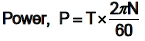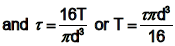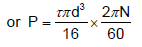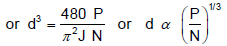QUESTION: 5

When a shaft transmits power through gears, the shaft experiences

Solution:
QUESTION: 6

A solid circular shaft is subjected to a bending moment M and twisting moment T. What is the equivalent twisting moment Te which will produce the same maximum shear stress as the above combination?

Solution: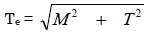QUESTION: 7

A member is subjected to the combined action of bending moment 400 Nm and torque 300 Nm. What respectively are the equivalent bending moment and equivalent torque?

Solution:

Equivalent BendingMoment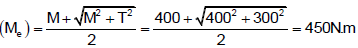Equivalent torque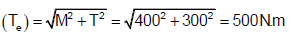QUESTION: 8

For obtaining the maximum shear stress induced in the shaft shown in the given figure, the torque should be equal to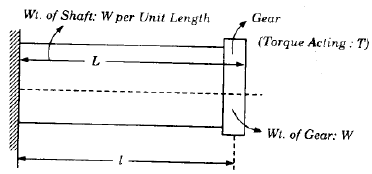Solution:

Bending Moment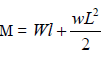QUESTION: 9

Which one of the following statements is correct? Shafts used in heavy duty speed reducers are generally subjected to:

Solution:
QUESTION: 10

A hollow shaft of outer dia 40 mm and inner dia of 20 mm is to be replaced by a solid shaft to transmit the same torque at the same maximum stress. What should be the diameter of the solid shaft?

Solution:

Section modules will be same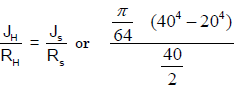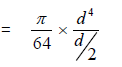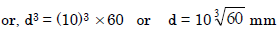QUESTION: 11

Two hollow shafts of the same material have the same length and outside diameter. Shaft 1 has internal diameter equal to one-third of the outer diameter and shaft 2 has internal diameter equal to half of the outer diameter. If both the shafts are subjected to the same torque, the ratio of their twists θ12 will be equal to:

Solution: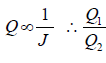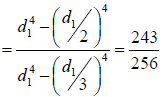QUESTION: 12

What is the total angle of twist of the stepped shaft subject to torque T shown in figure given above?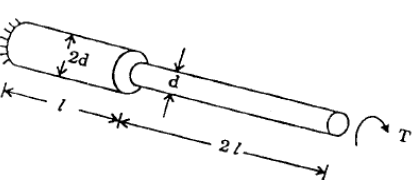Solution: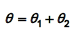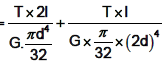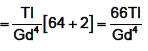QUESTION: 13

Two shafts are shown in the above figure. These two shafts will be torsionally equivalent to each other if their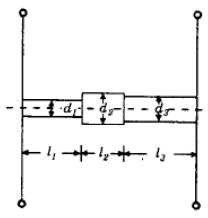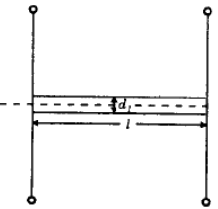Solution:
QUESTION: 14

The shear stress at a point in a shaft subjected to a torque is:

Solution: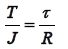QUESTION: 15

A hollow circular shaft having outside diameter 'D' and inside diameter ‟d‟ subjected to a constant twisting moment 'T' along its length. If the maximum shear stress produced in the shaft is σs then the twisting moment 'T' is given by:

Solution: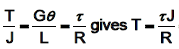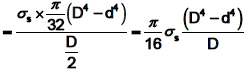QUESTION: 16

A hollow shaft of length L is fixed at its both ends. It is subjected to torque T at a  distance of  L/3 from one end. What is the reaction torque at the other end of the shaft?

Solution: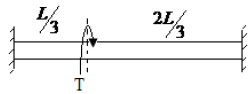QUESTION: 17

Two steel shafts, one solid of diameter D and the other hollow of outside diameter D and inside diameter D/2, are twisted to the same angle of twist per unit length. The ratio of maximum shear stress in solid shaft to that in the hollow shaft is:

Solution: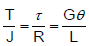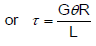as outside diameter of both the shaft is D so τ is same for both the cases.

QUESTION: 18

A stepped solid circular shaft shown in the given figure is built-in at its ends and is subjected to a torque To at the shoulder section. The ratio of reactive torque T1 and T2 at the ends is (J1 and J2 are polar moments of inertia):

Solution: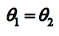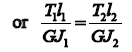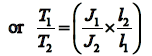QUESTION: 19

A steel shaft of outside diameter 100 mm is solid over one half of its length and hollow over the other half. Inside diameter of hollow portion is 50 mm. The shaft if held rigidly at two ends and a pulley is mounted at its midsection i.e., at the junction of solid and hollow portions. The shaft is twisted by applying torque on the pulley. If the torque carried by the solid portion of the shaft is 16000kg-m, then the torque carried by the hollow portion of the shaft will be:

Solution: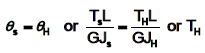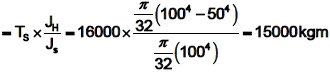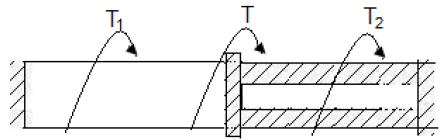QUESTION: 20

While transmitting the same power by a shaft, if its speed is doubled, what should be its new diameter if the maximum shear stress induced in the shaft remains same?

Solution: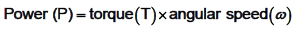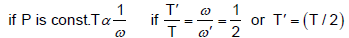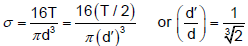QUESTION: 21

The equivalent bending moment under combined action of bending moment M and torque T is:

Solution:
QUESTION: 22

A shaft was initially subjected to bending moment and then was subjected to torsion. If the magnitude of bending moment is found to be the same as that of the torque, then the ratio of maximum bending stress to shear stress would be:

Solution:

Use equivalent bending moment formula,

1st case: Equivalent bending moment (Me) = M

2nd case: Equivalent bending moment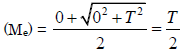QUESTION: 23

The ratio of torque carrying capacity of a solid shaft to that of a hollow shaft is given by: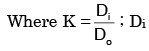= Inside diameter of hollow shaft and Do = Outside diameter of hollow
shaft. Shaft material is the same.

Solution:

τ should be same for both hollow and solid shaft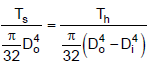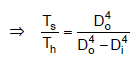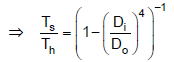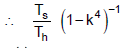QUESTION: 24

What is the maximum torque transmitted by a hollow shaft of external radius R and internal radius r?

Solution: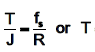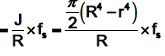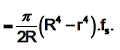QUESTION: 25

A circular section rod ABC is fixed at ends A and C. It is subjected to torque T at B. AB = BC = L and the polar moment of inertia of portions AB and BC are 2 J and J respectively. If G is the modulus of rigidity, what is the angle of twist at point B?

Solution: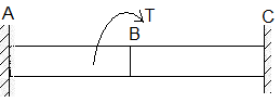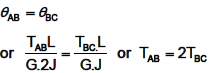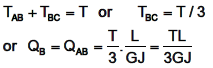QUESTION: 26

Assertion (A): In theory of torsion, shearing strains increase radically away from the longitudinal axis of the bar.

Reason (R): Plane transverse sections before loading remain plane after the torque is applied.

Solution:
QUESTION: 27

If two shafts of the same length, one of which is hollow, transmit equal torque and have equal maximum stress, then they should have equal.

Solution: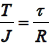Here T & τ are same, so J/R should be same i.e. polar modulus of section will be same.

QUESTION: 28

A shaft is subjected to a bending moment M = 400 N.m alld torque T = 300 N.m The equivalent bending moment is:

Solution: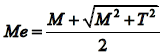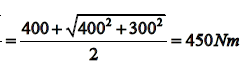QUESTION: 29

A solid shaft of diameter d is replaced by a hollow shaft of the same material and length.  The outside diameter of hollow shaft 2d/√3 while the inside diameter is d/√3 What is the ratio of the torsional stiffness of the hollow shaft to that of the solid shaft?

Solution:

Torsional stiffness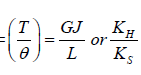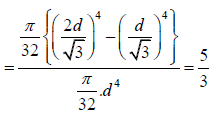QUESTION: 30

Two shafts having the same length and material are joined in series. If the ra tio of the diameter of the first shaft to that of the second shaft is 2, then the ratio of the angle of twist of the first shaft to that of the second shaft is:

Solution:

Angle of twist is proportional